# AlgebraIn this topic, we will look at

• Arithmetic and Geometric Sequences and Series
• Exponents and Logarithms
• The Binomial Theorem
• Solving Systems of Linear Equations
• Permutations and Combinations
• Proof by Induction
• Complex Number

•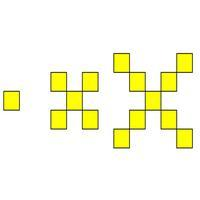#### Arithmetic Sequences

These are sequences where you go from term to term by adding a common difference. The sequence in the image 1, 5 , 9 has a common difference of 4 since we add 4 to the previous term. There are formula in the booklet to help you with this...

•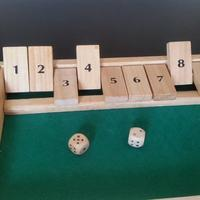#### Geometric Sequences

These are sequence where you go from term to term by multiplying by a common ratio. The sequence in the image 1, 2 , 4 , 8 has a common ration of 2 since we multiply the previous term by 2. There are formula in the booklet to help you with this topic, but

•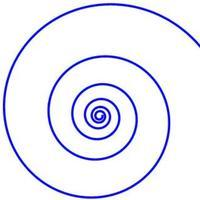#### Indices and Logarithms

Logarithms are useful for solving problems involving indices (or exponents). In fact, logarithms are just indices in disguise! The definition of a logarithm helps you to see its equivalence with indices

•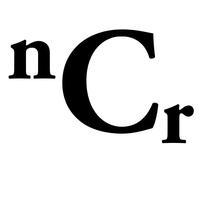#### Counting Principles

This page deals with all the counting principles in the HL course: Arrangements, Permutations and Combinations. You need to be familiar with combinations for the Binomial Theorem, but it is also useful in its own right to be able to work out, for example,

•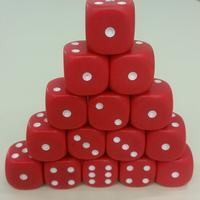#### Binomial Theorem SL

The following page will help you with questions about the Binomial Theorem (or Binomial Expansion). The Binomial Theorem is used for expanding brackets in the form (a + b)n . Questions on this topic are usually short ones: you usually only have to find one

•#### Binomial Theorem HL

The following page will help you with questions about the Binomial Theorem (or Binomial Expansion). The Binomial Theorem is used for expanding brackets in the form (a + b)n . Questions on this topic are usually short ones: you usually only have to find one

•#### Deductive Proofs

On this page, we will look at deductive reasoning in order to be able to make direct proofs. This is a hugely important topic in mathematics, since we like to be absolutely sure of the results we have found. However, this can be a challenging - when a prob

•"...when you have eliminated the impossible, whatever remains, however improbable, must be the truth?" Sherlock Holmes in The Sign of the Four by Sir Arthur Conan Doyle. Holmes was a wonderful detective and he would also have been excellent at carrying out

•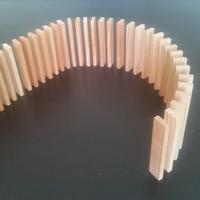#### Proof by Induction

Proof by Induction is a method of proof commonly used in HL mathematics. The method is always the same and questions are worth a good deal of marks in an exam. Therefore, it is really worth investing time to understand how to use it! Questions involving s

•#### Complex Numbers - The Basics

This page will allow you to become confident in the basic principles of complex numbers. It is important to understand, and be able to use, the three different forms of a complex number: Cartesian, Polar and Euler. You will learn about the Argand diagram,

•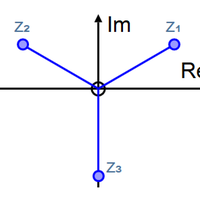#### Complex Numbers - de Moivre's Theorem

De Moivre's Theorem gives a formula for calculating complex numbers. It enables us to connect complex numbers and trigonometry. Most importantly, it is incredibly useful for finding powers and roots of complex numbers. It can be stated in a number of ways:

•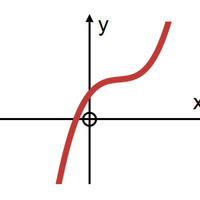#### Complex Numbers - Roots of Polynomials

The Conjugate Root Theorem states that if the complex number a + ib is a root of a polynomial in one variable with real coefficients, then the complex conjugate a - bi also a root of that polynomial. This is a useful theorem for solving polynomials with re